📢 大家好，我是小丞同学，本文主要写 JavaScript 中的 7 种继承方式，深入理解每种方式所存在的问题同时新的方式是如何解决问题的

📢 非常感谢你的阅读，不对的地方欢迎指正 🙏

📢

## 💌 前言 ​

“实现继承是 `ECMAScript` 唯一支持的继承方式，且这主要通过原型链来实现。”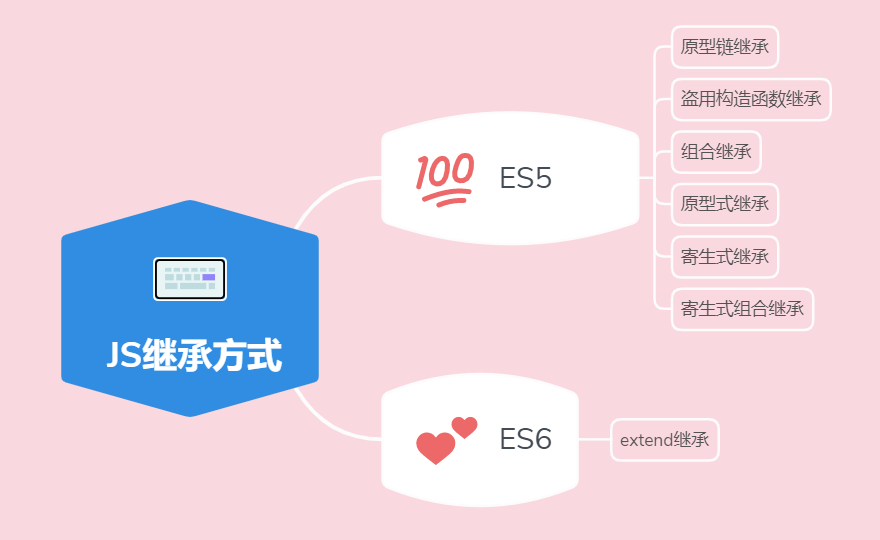## 💟息息相关的 6 种继承方式 ​

### 🍏 一、原型链继承 ​

#### 2. 实现方法 ​

js
``````// 定义父类函数
function Father() {
// 定义父类属性
this.name = 'father'
}
// 给父类的原型添加方法
Father.prototype.say = function () {
console.log('我是爸爸');
}
// 创建子类函数
function Son() {}
// 实现继承
Son.prototype = new Father()
// 打印参考
console.log(Son.prototype) // Father {name: "father"}``````

1. 创建一个空对象
2. 继承函数原型，将这个新对象的 `__proto__` 属性赋值为构造函数的原型对象
3. 构造函数内部的 `this` 指向新对象
4. 执行函数体
5. 返回这个新对象

#### 3. 存在的问题 ​

js
``````function Father() {
// 定义父类属性为引用数据类型
this.a = [1, 2, 3, 4]
}``````

js
``````let son1 = new Son()
let son2 = new Son()``````

js
``son1.a.push(5)``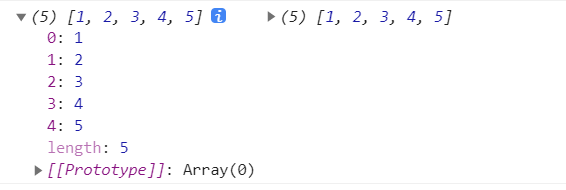#### 4. 优点与不足 ​

• 父类的方法可以复用
• 操作简单
##### 缺点 ​
• 对于引用数据类型数据会被子类共享，也就是改一个其他都会改
• 创建子类实例时，无法向父类构造函数传参，不够灵活。

### 🍉 二、盗用构造函数继承 ​

#### 1. 基本思想 ​

js
``````function Son() {
this.a = [1, 2, 3, 4]
}``````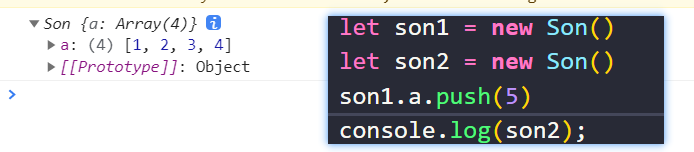#### 2. 实现方法 ​

js
``````function Father() {
this.a = [1, 2, 3, 4]
}

function Son() {
Father.call(this)
}
let son1 = new Son()
let son2 = new Son()
son1.a.push(5)
console.log(son1, son2)``````

#### 3. 存在的问题 ​

js
``````Father.prototype.say = function () {
console.log(111);
}``````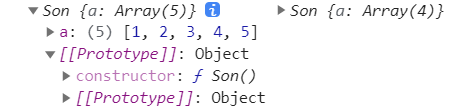#### 4. 优点与不足 ​

##### 优点： ​
• 解决了无法共享引用值的问题
• 能够传递参数
##### 缺点： ​
• 只能继承父类的实例属性和方法，不能继承父类的原型属性和方法
• 父类方法无法复用。每次实例化子类，都要执行父类函数。重新声明父类所定义的方法，无法复用。

### 🍊 三、组合继承 ​

#### 2. 实现方法 ​

js
``````function Father() {
this.a = [1, 2, 3, 4]
}
Father.prototype.say = function () {
console.log(111);
}
function Son() {
Father.call(this)
}
Son.prototype = new Father()
let son1 = new Son()
let son2 = new Son()``````

js
``Son.prototype = new Father()``

#### 3. 存在的问题 ​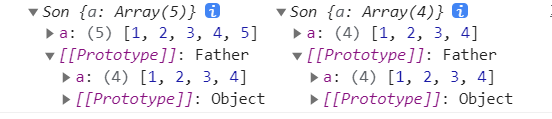`Father` 自身的属性手动添加到了 `Son` 的身上，因此在 `Son` 实例化出来的对象上，会有一个 `a` 属性，原型上也会有一个 `a` 属性

1. `new` 的时候
2. `call` 的时候

#### 4. 优点和不足 ​

##### 优点： ​
• 解决原型链继承中属性被共享的问题
• 解决借用构造函数解决不能继承父类原型对象的问题
##### 缺点： ​
• 调用了两次的父类函数，有性能问题
• 由于两次调用，会造成实例和原型上有相同的属性或方法

### 🍋 四、原型式继承 ​

#### 2. 实现方法 ​

js
``````function object(obj) {
function F(){};
// 将对象赋值给构造函数的原型
F.prototype = obj;
// 返回 new 期间创建的新对象
return new F();
}``````

js
``````let student = {name:'xxx'}
let another = object(student)``````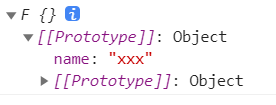#### 3. 存在的问题 ​

js
``````let student = {name:['ljc']}
let one = object(student)
let two = object(student)
one.name.push('aaa')
two.name.push('bbb')``````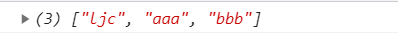#### 4. 优点和不足 ​

• 兼容性好，简单
• 不需要单独创建构造函数
##### 缺点： ​
• 多个实例共享被继承的属性，存在被篡改的情况
• 不能传递参数

`ES5` 中增加的 `Object.create()` 的方法，能够代替上面的 `object` 方法。也为原型式继承提供了规范

### 🍌 五、寄生式继承 ​

#### 2. 实现方法 ​

js
``````function createAnother(original) {
let clone = object(original); // 继承一个对象 返回新函数
clone.sayHi = function () {
console.log('hi');
};
return clone; // 返回这个对象
}``````

#### 3. 优点和不足 ​

##### 优点： ​
• 只需要关注对象本身，不在乎类型和构造函数的场景
##### 缺点： ​
• 函数难以重用
• 多个实例共享被继承的属性，存在被篡改的情况
• 无法传递参数

### 🍑 六、寄生式组合继承 ​

#### 2. 实现方法 ​

js
``````function Father() {
this.a = [1, 2, 3, 4]
}
Father.prototype.say = function () {
console.log(111);
}
function Son() {
Father.call(this)
}
Son.prototype = Object.create(Father)
let son1 = new Son()
let son2 = new Son()``````#### 3. 存在的问题 ​

js
``````Son.prototype.sayHi = function() {
console.log('Hi')
}``````#### 4. 优点和不足 ​

##### 优点： ​
• 基本上是最佳的继承方案了，当然还有圣杯继承

• 只调用了父类构造函数一次，节约了性能。

• 避免生成了不必要的属性

• 子类原型被重写

## ES6 中的继承🎭 ​

js
``````class Father {}
class Son extends Father {
constructor() {
super()
}
}``````## 参考文献 ​

《JavaScript 高级程序设计》

《JavaScript常见八种继承方案》

Last updated: